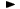ADVFN HomeHelpFinancialsDeeper AnalysisFINANCIAL RATIOS - Liquidity RatiosLiquidity Ratio

# Liquidity Ratio

Liquidity ratio, expresses a company's ability to repay short-term creditors out of its total cash. The liquidity ratio is the result of dividing the total cash by short-term borrowings. It shows the number of times short-term liabilities are covered by cash. If the value is greater than 1.00, it means fully covered.

The formula is the following:

= cash & equivalents / creditors,short